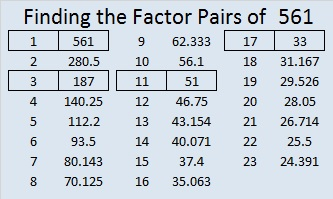# 561 gives a false positive to these 102 prime number tests

We can use some easy divisibility tests to find two of the factors of 561.

• 5 + 6 + 1 = 12, a multiple of 3 so 561 is divisible by 3.
• 5 – 6 + 1 = 0, which is divisible by 11 so 561 can be evenly divided by 11.

But if we only do those divisibility tests, we will miss something very significant about the number 561:

If you divide 2^561 by 561, the remainder will be 2. When we are more interested in the remainder than the quotient, we can simply type “2, x^y, 561, Mod, 561, =” into the computer’s scientific calculator:This is only a picture of a calculator.

2^561 (mod 561) = 2 means that 561 is VERY LIKELY a prime number, but this is one time when VERY LIKELY does not mean ACTUALLY!

561 has something in common with the number 341. Yes, both of them pass this quick prime number test, and both of them are composite numbers divisible by 11. Both numbers are called pseudo-prime numbers. (341 and 561 are the two smallest composite numbers to give a false positive to this particular test.)

561 is even more remarkable than 341:

• 2^561 (mod 561) = 2, and 2^341 (mod 341) = 2 (Both numbers pass.)
• 3^561 (mod 561) = 3, while 3^341 (mod 341) = 168 (561 passes; 341 fails.)
• 5^561 (mod 561) = 5
• 7^561 (mod 561) = 7
• 11^561 (mod 561) = 11
• 13^561 (mod 561) = 13
• 17^561 (mod 561) = 17
• etc.

There are 102 prime numbers less than 561, and p^561 (mod 561) = p for every single one of them! 561 acts like a prime number in those 102 ways.

In 1910 R. D. Carmichael discovered that 561 is the first COMPOSITE number that passes ALL those modular (remainder) prime number tests, so 561 is the first Carmichael number. Yes, there will be more – in fact, infinitely more.

R. D. Carmichael actually found that 561 passes ALL 559 prime number tests using each whole number between 1 and 561, for example 33^561 (mod 561) = 33. All prime numbers can make a similar claim, but 561 is the smallest composite number with that property.

(Note: I did not use the standard mathematical notation for this property, but what I used is equivalent to it and doesn’t require parenthesis when typing it into the computer’s scientific calculator. Also I think “=” is less intimidating looking for some of my readers than “≡”.)

There are other reasons why the number 561 is an interesting number:

Because 33 x 34/2 = 561, we know that 561 is the 33rd triangular number and is equal to 1 + 2 + 3 + . . . + 31 + 32 + 33, the sum of the first 33 whole numbers.

Because 17 x (2 x 17 – 1) = 561, we know that 561 is the 17th hexagonal number. (All hexagonal numbers are also triangular numbers.)

561 is also the hypotenuse of the Pythagorean triple 264-495-561. What is the greatest common factor of those three numbers? Hint: it is one of the factors of 561 listed below:

——————————————————————–

• 561 is a composite number.
• Prime factorization: 561 = 3 x 11 x 17
• The exponents in the prime factorization are 1, 1, and 1. Adding one to each and multiplying we get (1 + 1)(1 + 1)(1 + 1) = 2 x 2 x 2 = 8. Therefore 561 has exactly 8 factors.
• Factors of 561: 1, 3, 11, 17, 33, 51, 187, 561
• Factor pairs: 561 = 1 x 561, 3 x 187, 11 x 51, or 17 x 33
• 561 has no square factors that allow its square root to be simplified. √561 ≈ 23.6854Advertisements